### Home > GB8I > Chapter 5 Unit 6 > Lesson INT1: 5.1.1 > Problem5-6

5-6.
1. What if the data for Lennie and George (from problem 5‑1) matched the data in each table below? Assuming that the growth of the rabbits multiplies as it did in problem 5‑1, complete each of the following tables. Show your thinking or give a brief explanation of how you know what the missing entries are. Homework Help ✎

2. Homework Help ✎

 a.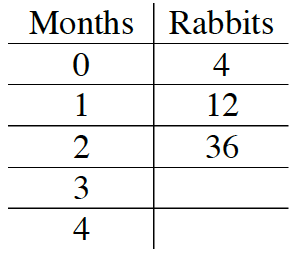b.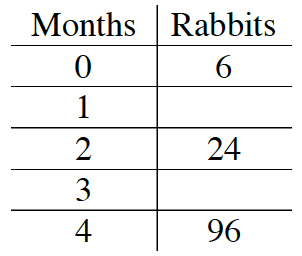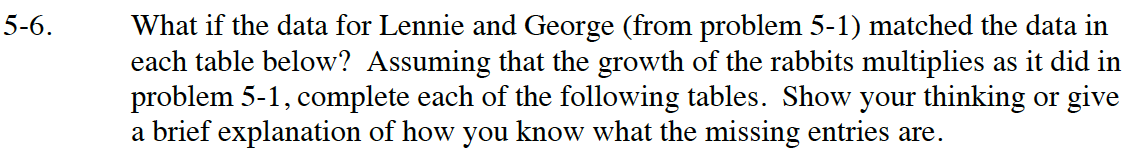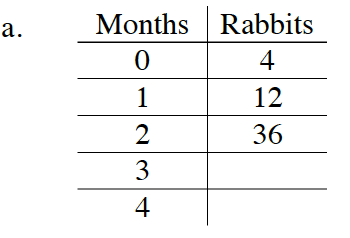What could be the multiplier? Check it with given values.

Use the multiplier to determine the missing values.

3 Months = 108 Rabbits;
4 Months = 324 Rabbits.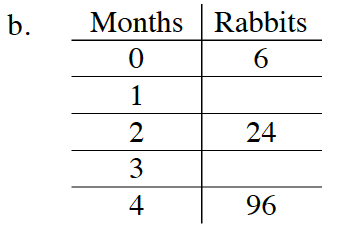To find the multiplier, think about what happens when you multiply by 2.

Use the multiplier to determine the missing values.

1 Month = 12 Rabbits
3 Months = 48 Rabbits